# How to Custom Sort in SQL ORDER BY Clause?

• Last Updated : 28 Oct, 2021

By default SQL ORDER BY sort, the column in ascending order but when the descending order is needed ORDER BY DESC can be used. In case when we need a custom sort then we need to use a CASE statement where we have to mention the priorities to get the column sorted.

In this article let us see how we can custom sort in a table using order by using MSSQL as a server.

Syntax:

```SELECT * FROM table_name
ORDER BY CASE
WHEN column_field = "value1" THEN priority1
WHEN column_field = "value2" THEN priority2
WHEN column_field = "value3" THEN priority3
.
ELSE priorityn
END ASC```

Step 1: We are creating a Database. For this use the below command to create a database named GeeksforGeeks.

Query:

`CREATE DATABASE GeeksforGeeks;`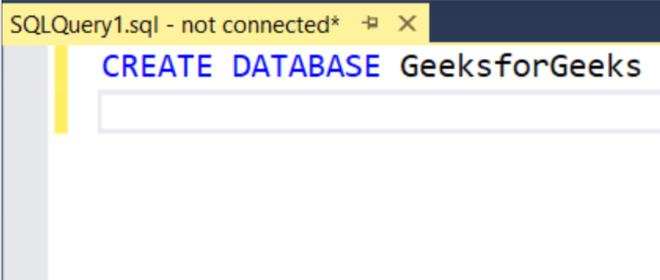Step 2: To use the GeeksforGeeks database use the below command.

Query:

`USE GeeksforGeeks`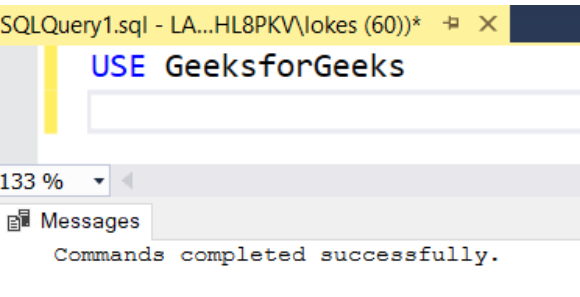Step 3: Now we are creating a table. Create a table student_marks with 4 columns using the following SQL query.

Query:

```CREATE TABLE student_marks(
stu_id VARCHAR(20),
stu_name VARCHAR(20),
stu_branch VARCHAR(20),
total_marks INT
)```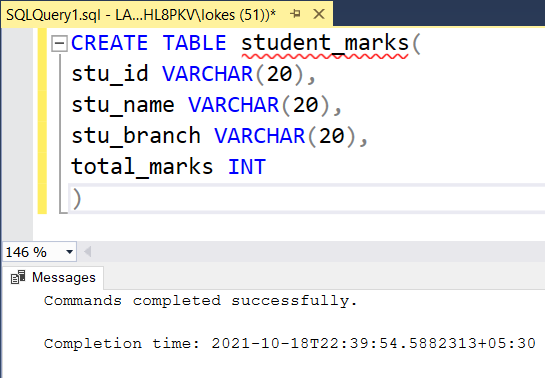Step 4: View the description of the table.

Query:

`EXEC sp_columns student_marks`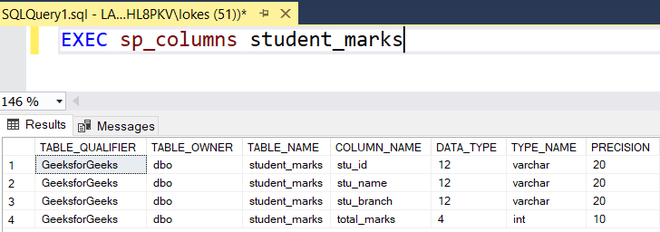Step 5: The query for Inserting rows into the Table. Insert rows into the student_marks  table using the following SQL query.

Query:

```INSERT INTO student_marks
( '1002','KIRAN','E.C.E', 540),
( '5001','PRANAV','I.C.E', 450),
( '2002','SRUTHI','C.S.E', 480),
( '2003','HARSITHA','C.S.E', 534),
( '3001','SAI','I.T', 560),
( '3002','HARSH','I.T', 510),
( '4001','HARSHINI','E.E.E', 500) ```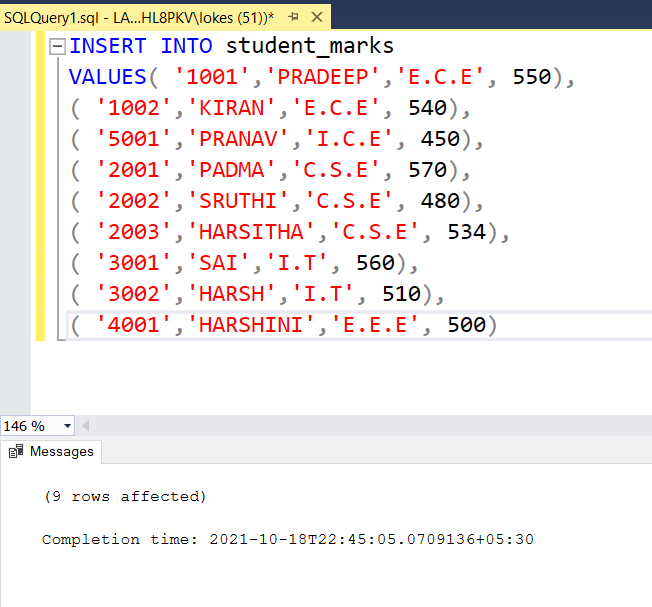Step 6: Viewing the inserted data

Query:

`SELECT * FROM student_marks`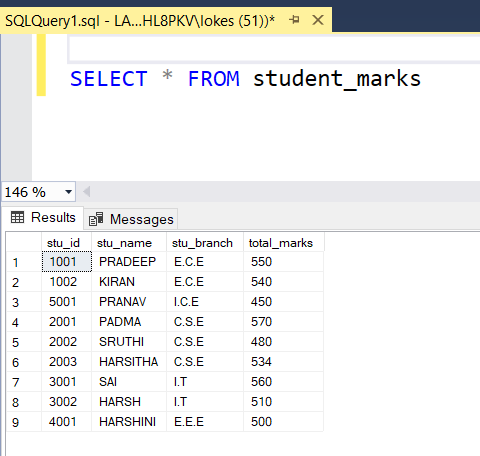• Query to custom sort based on branch order of C.S.E, I.T, E.C.E, I.C.E, E.E.E also show the difference between the default sort using ORDER BY.

Default sort by ORDER BY :

```SELECT * FROM student_marks
ORDER BY stu_branch ```

Output: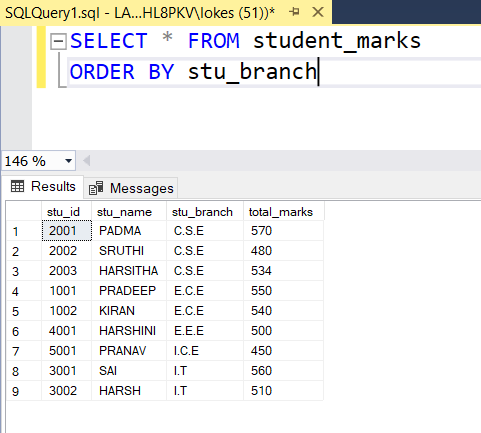Custom sort by ORDER BY:

```SELECT * FROM student_marks
ORDER BY CASE
WHEN stu_branch = 'C.S.E' then 1
WHEN stu_branch = 'I.T' then 2
WHEN stu_branch = 'E.C.E' then 3
WHEN stu_branch = 'I.C.E' then 4
WHEN stu_branch = 'E.E.E' then 5
END ASC  ```

Output: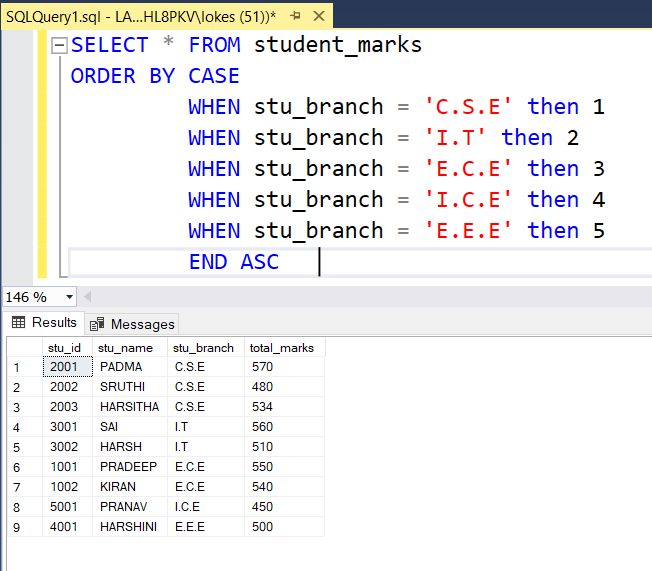My Personal Notes arrow_drop_up